Courses

# Figure Matrix Quiz I, Non Verbal Reasoning

## 15 Questions MCQ Test Non Verbal Reasoning | Figure Matrix Quiz I, Non Verbal Reasoning

Description
This mock test of Figure Matrix Quiz I, Non Verbal Reasoning for LR helps you for every LR entrance exam. This contains 15 Multiple Choice Questions for LR Figure Matrix Quiz I, Non Verbal Reasoning (mcq) to study with solutions a complete question bank. The solved questions answers in this Figure Matrix Quiz I, Non Verbal Reasoning quiz give you a good mix of easy questions and tough questions. LR students definitely take this Figure Matrix Quiz I, Non Verbal Reasoning exercise for a better result in the exam. You can find other Figure Matrix Quiz I, Non Verbal Reasoning extra questions, long questions & short questions for LR on EduRev as well by searching above.
QUESTION: 1

### Directions to Solve In each of the following questions, find out which of the answer figures (1), (2), (3) and (4) completes the figure matrix ? Question - Select a suitable figure from the four alternatives that would complete the figure matrix.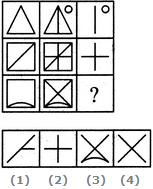Solution: The third figure in each row comprises of parts which are not common to the first two figures.
QUESTION: 2

### Directions to Solve In each of the following questions, find out which of the answer figures (1), (2), (3) and (4) completes the figure matrix ? Question -   Select a suitable figure from the four alternatives that would complete the figure matrix.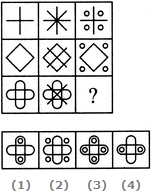Solution:

In each row, the second figure is obtained from the first figure by adding two mutually perpendicular line segments at the centre and the third figure is obtained from the first figure by adding four circles outside the main figure.

QUESTION: 3

### Directions to Solve In each of the following questions, find out which of the answer figures (1), (2), (3) and (4) completes the figure matrix ? Question -   Select a suitable figure from the four alternatives that would complete the figure matrix.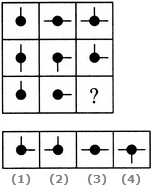Solution:

In each row, the third figure comprises of a black circle and only those line segments which are not common to the first and the second figures.

From the first row we see that the third picture is a composition of the first two.
For the second row, the same pattern follows on, but we remove the line that is common to both the pictures, which is the bottom one.
For the third row, there are no common pictures, so it's just a simple composition picture of the first two.

QUESTION: 4

In each of the following questions, find out which of the answer figures (1), (2), (3) and (4) completes the figure matrix ?

Question -

Select a suitable figure from the four alternatives that would complete the figure matrix.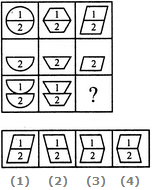Solution: In each column, the second figure (middle figure) is obtained by removing the upper part of the first figure (uppermost figure) and the third figure (lowermost figure) is obtained by vertically inverting the upper part of the first figure.
QUESTION: 5

In each of the following questions, find out which of the answer figures (1), (2), (3) and (4) completes the figure matrix ?

Question -

Select a suitable figure from the four alternatives that would complete the figure matrix.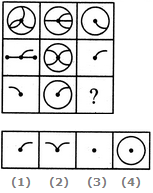Solution: The third figure in each row comprises of the parts common to the first two figures.
QUESTION: 6

In each of the following questions, find out which of the answer figures (1), (2), (3) and (4) completes the figure matrix ?

Question -

Select a suitable figure from the four alternatives that would complete the figure matrix.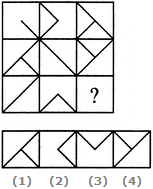Solution: The third figure in each row comprises of parts which are not common to the first two figures.
QUESTION: 7

In each of the following questions, find out which of the answer figures (1), (2), (3) and (4) completes the figure matrix ?

Question -

Select a suitable figure from the four alternatives that would complete the figure matrix.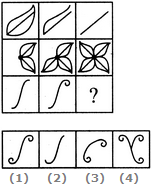Solution: The number of components in each row either increases or decreases from left to right. In the third row, it increases.
QUESTION: 8

In each of the following questions, find out which of the answer figures (1), (2), (3) and (4) completes the figure matrix ?

Question -

Select a suitable figure from the four alternatives that would complete the figure matrix.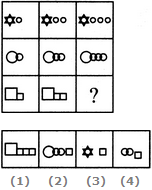Solution: In each row, the second figure is obtained from the first figure by increasing the number of smaller elements by one and the third figure is obtained from the second figure by increasing the number of smaller elements by one.
QUESTION: 9

In each of the following questions, find out which of the answer figures (1), (2), (3) and (4) completes the figure matrix ?

Question -

Select a suitable figure from the four alternatives that would complete the figure matrix.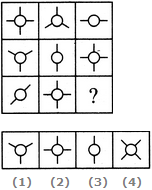Solution: Each row (as well as each column) contains a figure consisting of a circle and two line segments, a figure consisting of a circle and three line segments and a figure consisting of a circle and four line segments.
QUESTION: 10

In each of the following questions, find out which of the answer figures (1), (2), (3) and (4) completes the figure matrix ?

Question -

Select a suitable figure from the four alternatives that would complete the figure matrix.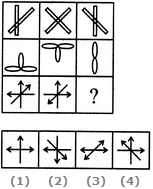Solution: The third figure in each row comprises of parts which are not common to the first two figures.
QUESTION: 11

In each of the following questions, find out which of the answer figures (1), (2), (3) and (4) completes the figure matrix ?

Question -

Select a suitable figure from the four alternatives that would complete the figure matrix.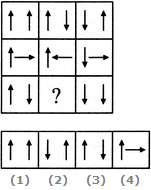Solution: In each row, the second figure is obtained from the first figure by reversing the direction of the RHS arrow and the third figure is obtained from the second figure by reversing the direction of both the arrows.
QUESTION: 12

In each of the following questions, find out which of the answer figures (1), (2), (3) and (4) completes the figure matrix ?

Question -

Select a suitable figure from the four alternatives that would complete the figure matrix.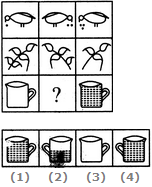Solution: In each row, the figures are getting laterally inverted in each step. The number of components or the quantities are either increasing or decreasing from left to right sequentially.
QUESTION: 13

In each of the following questions, find out which of the answer figures (1), (2), (3) and (4) completes the figure matrix ?

Question -

Select a suitable figure from the four alternatives that would complete the figure matrix.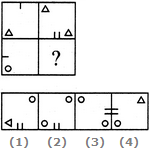Solution: The second figure is obtained from the first figure by moving the line segment to the opposite side of the square boundary and replacing it with two similar line segments. Also, the element in the lower-left corner gets replaced by two similar elements - one placed in the upper-left and the other placed in the lower-right corner.
QUESTION: 14

In each of the following questions, find out which of the answer figures (1), (2), (3) and (4) completes the figure matrix ?

Question -

Select a suitable figure from the four alternatives that would complete the figure matrix.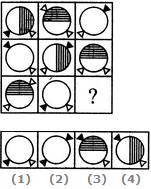Solution: In each row, there are 3 types of shadings of circles - a circle is unshaded, another circle has its right half shaded with vertical lines and yet another circle has its upper half shaded with horizontal lines. There are three specified positions of the two triangles each of which is used only once in a row. Also, two of the figures in each row have one triangle shaded.
QUESTION: 15

In each of the following questions, find out which of the answer figures (1), (2), (3) and (4) completes the figure matrix ?

Question -

Select a suitable figure from the four alternatives that would complete the figure matrix.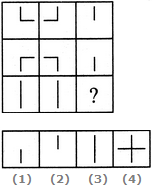Solution: In each row, the third figure is a collection of the common elements (line segments) of the first and the second figures.

Track your progress, build streaks, highlight & save important lessons and more!

### Similar Content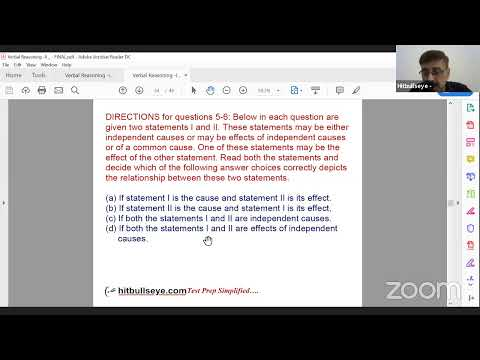### Related tests